Extensive form games

# 1 Getting Going

First open R or Rstudio and install the hop package from github (make sure you have devtools installed to be able to run the below command):

devtools::install_github("macartan/hop")

library(hop)

# 2 Game trees

The gt_tree function graphs game trees given a full specification of an extensive form game with complete information. To define an extensive form game we need to define:

• $$H$$: a set of histories – that is all the possible paths that can be followed
• $$P$$: a player function that says what player moves at what point in each history
• $$U$$: Utilities awarded to players at the end of each history

## 2.1 Illustration 1; Sequential Chicken

Here is a simple example. Lets imagine a Chicken game played out over time. H, P and U, are defined as follows:

H <- matrix(c("0", "0", "0", "0", "C", "C", "D", "D", "C", "D",  "C", "D"),4)
P <- matrix(c(rep(1,4), rep(2,4)),4)
U <- matrix(c(1,0,2,-1,1,2, 0, -1), 4)

Then the game tree looks like this:

gt_tree(H,U,P, title = "Sequential Chicken", solution = TRUE)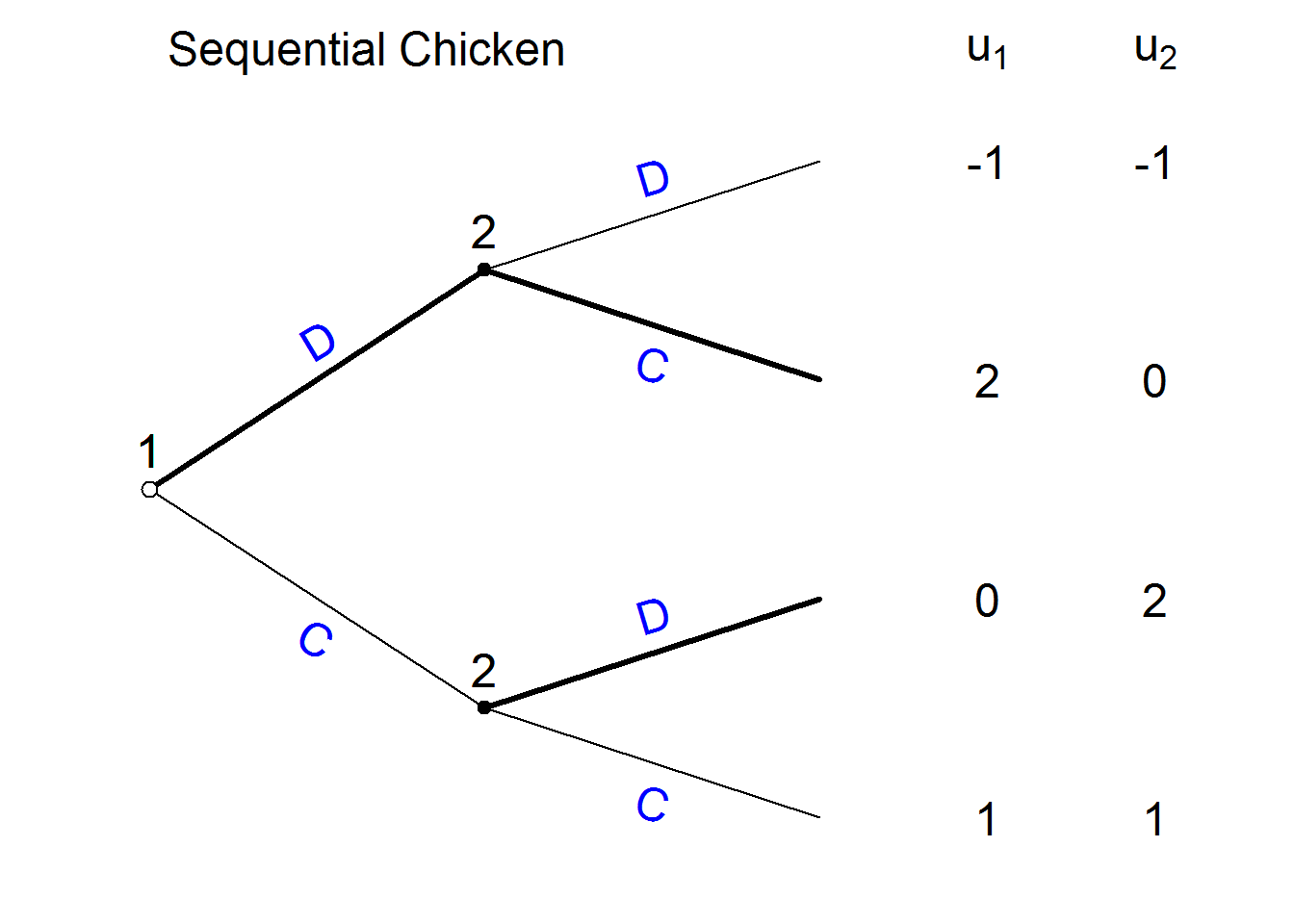Note that gt_tree interprets the set of histories as a tree; it figures out the branches based on when the histories depart from each other and the nodes from the player function. The underlying data looks like this:

History:

rownames(H) <- c("H1", "H2", "H3", "H4")
kable(H, col.names = c("T0", "T1", "T2"))
T0 T1 T2
H1 0 C C
H2 0 C D
H3 0 D C
H4 0 D D

Player Function:

rownames(P) <- c("H1", "H2", "H3", "H4")
kable(P, col.names = c("T1", "T2"))
T1 T2
H1 1 2
H2 1 2
H3 1 2
H4 1 2

Utilities:

rownames(U) <- c("H1", "H2", "H3", "H4")
kable(U, col.names =c("U1", "U2"))
U1 U2
H1 1 1
H2 0 2
H3 2 0
H4 -1 -1

## 2.2 Illustration 2: The Hostage Game

The introductory text describes a hostage game in which options depend on past history.

Here is the graph with the solution:

gt_tree(
H = matrix(c("Take hostages", "Take hostages", "Don't take", "Pay Ransom", "Don't pay", ""),3),
U = matrix(c(1,-1,0,-1,-2,0), 3),
P = matrix(c(rep(1,3), c(2,2,1)),3),
solution=TRUE,
player.names=c("Militants", "Gov")
)
##  "Initial history column added"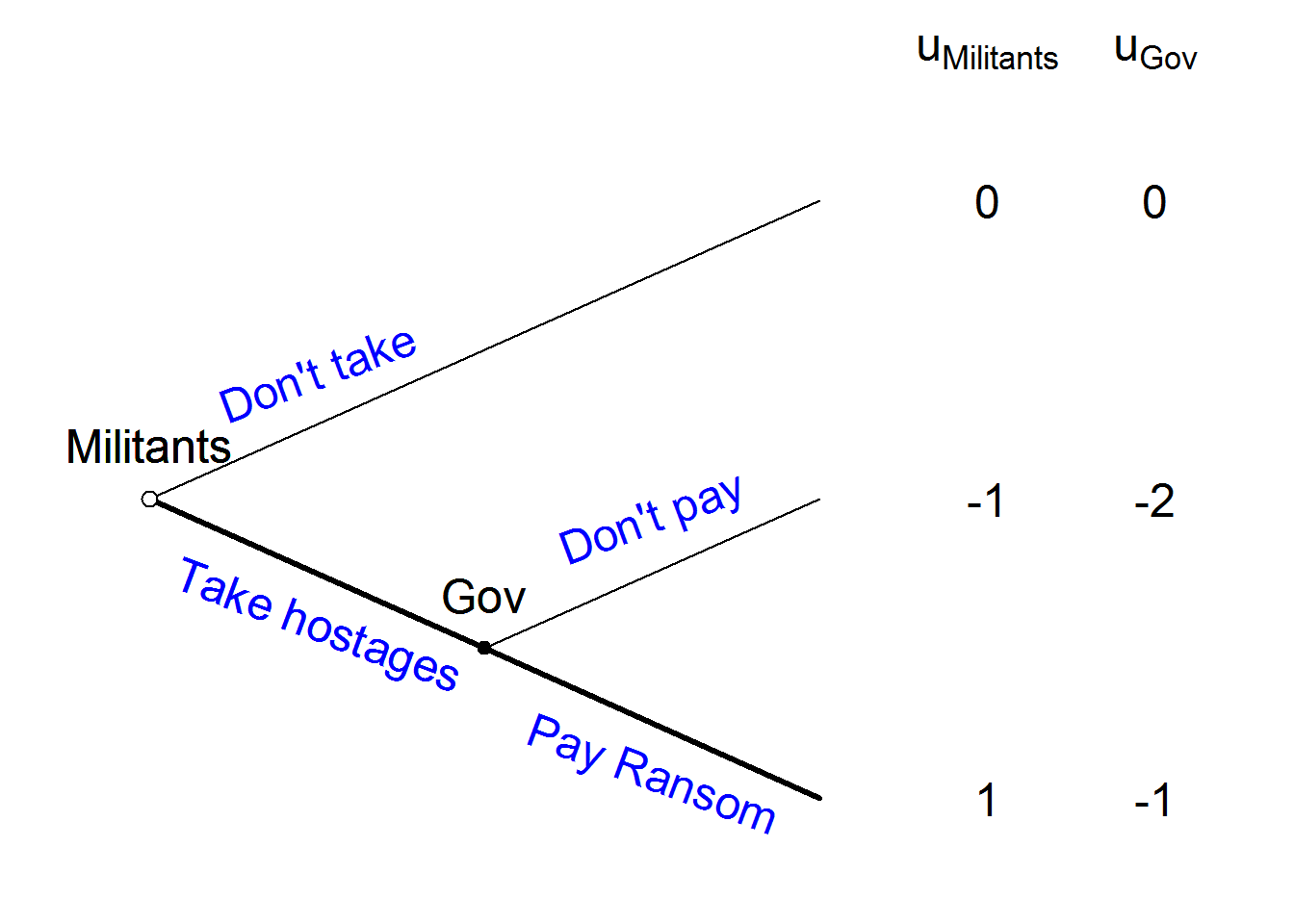# 3 More complex games

The function can allow much more complex games; the key chllenge is to be able to write out all the histories. Somestimes these histories can be generated in an automated way to make families of more complex games.

centipede <- function(n, ...){
H <- matrix("C", n+1, n+1)
H[lower.tri(H)] <- "D"
H <- H[,n:1]
U <- cbind(U1 = ((n+1):1) + 2*((n+1):1)%%2 - 2,
U2 = ((n+1):1) - 2*((n+1):1)%%2 + 1)
P <-  t(replicate(n+1, rev(rep(1:2, length = n+1))))
P[lower.tri(P)] <- t(P)[lower.tri(P)]
P <- t(P)[,(n+1):1]
gt_tree(H,U,P, ...)
}

Now use this to graph a short and a long centipede game:

centipede(n = 3, solution = TRUE)       
##  "Initial history column added"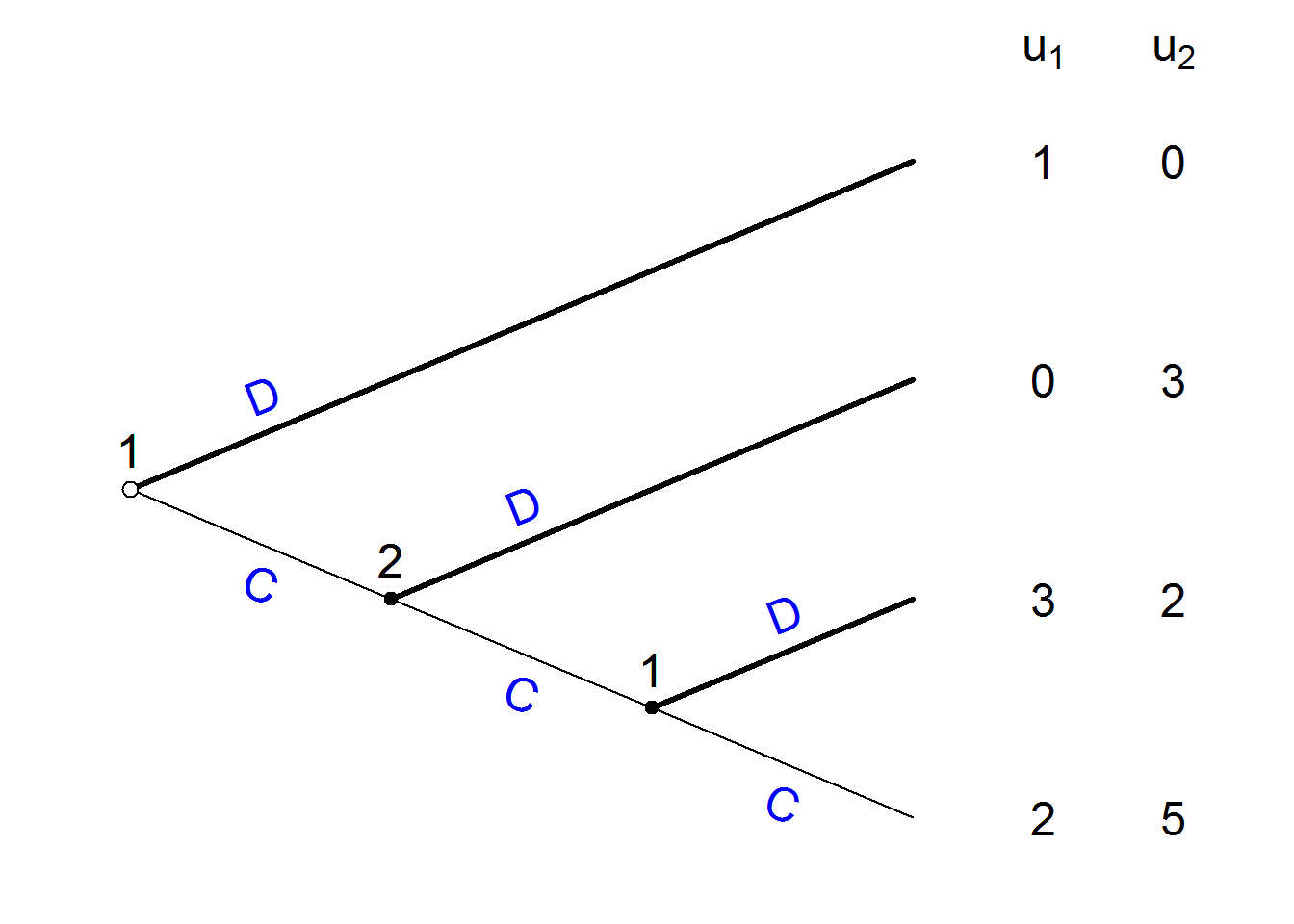centipede(n = 8, solution = TRUE, textsize=.75)     
##  "Initial history column added"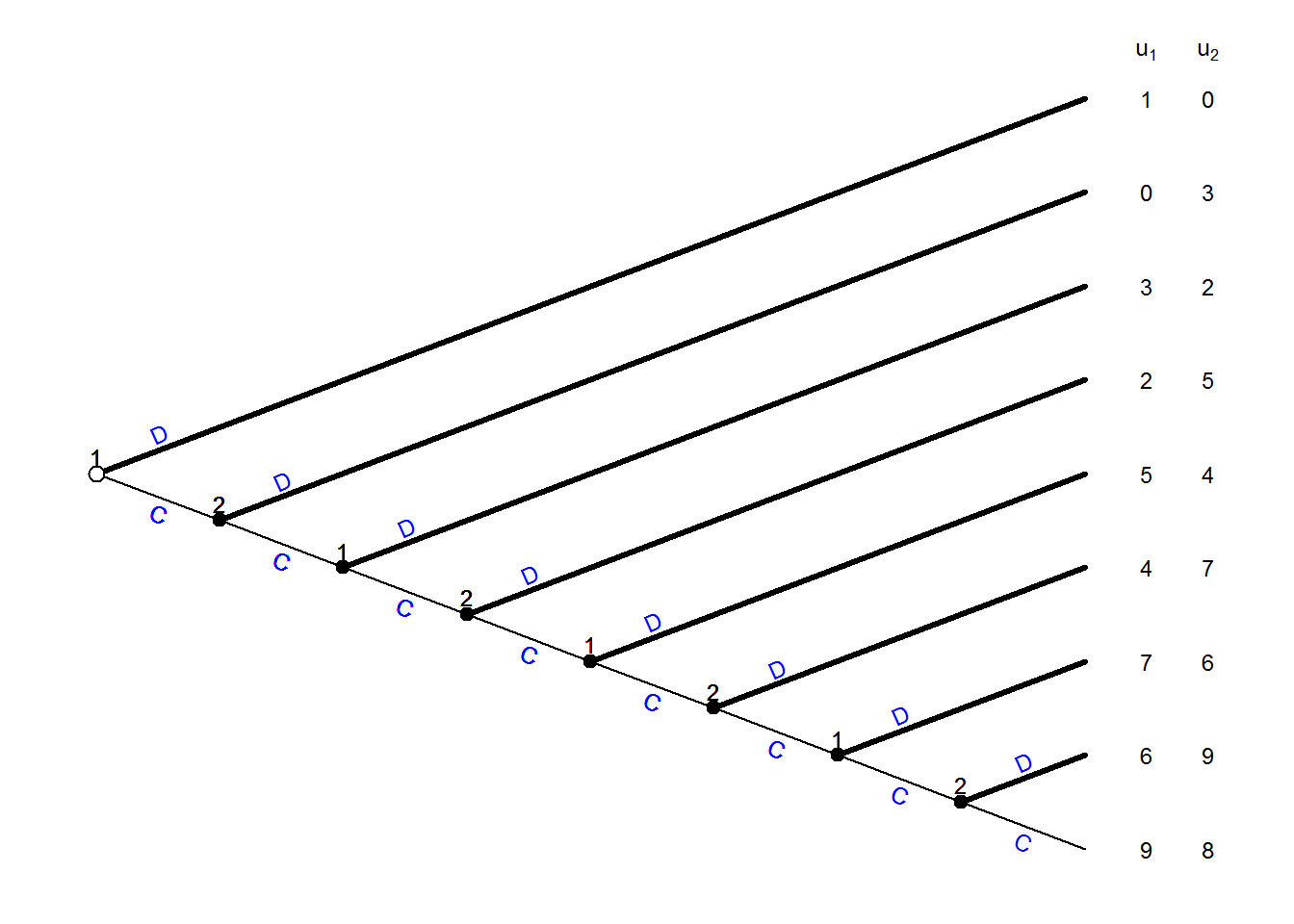# 4 Adding and forcing solutions

You can add or remove solutions using the solution argument. The sequential chicken without a solution is drawn like this:

gt_tree(H,U,P, title = "Sequential Chicken", solution = FALSE)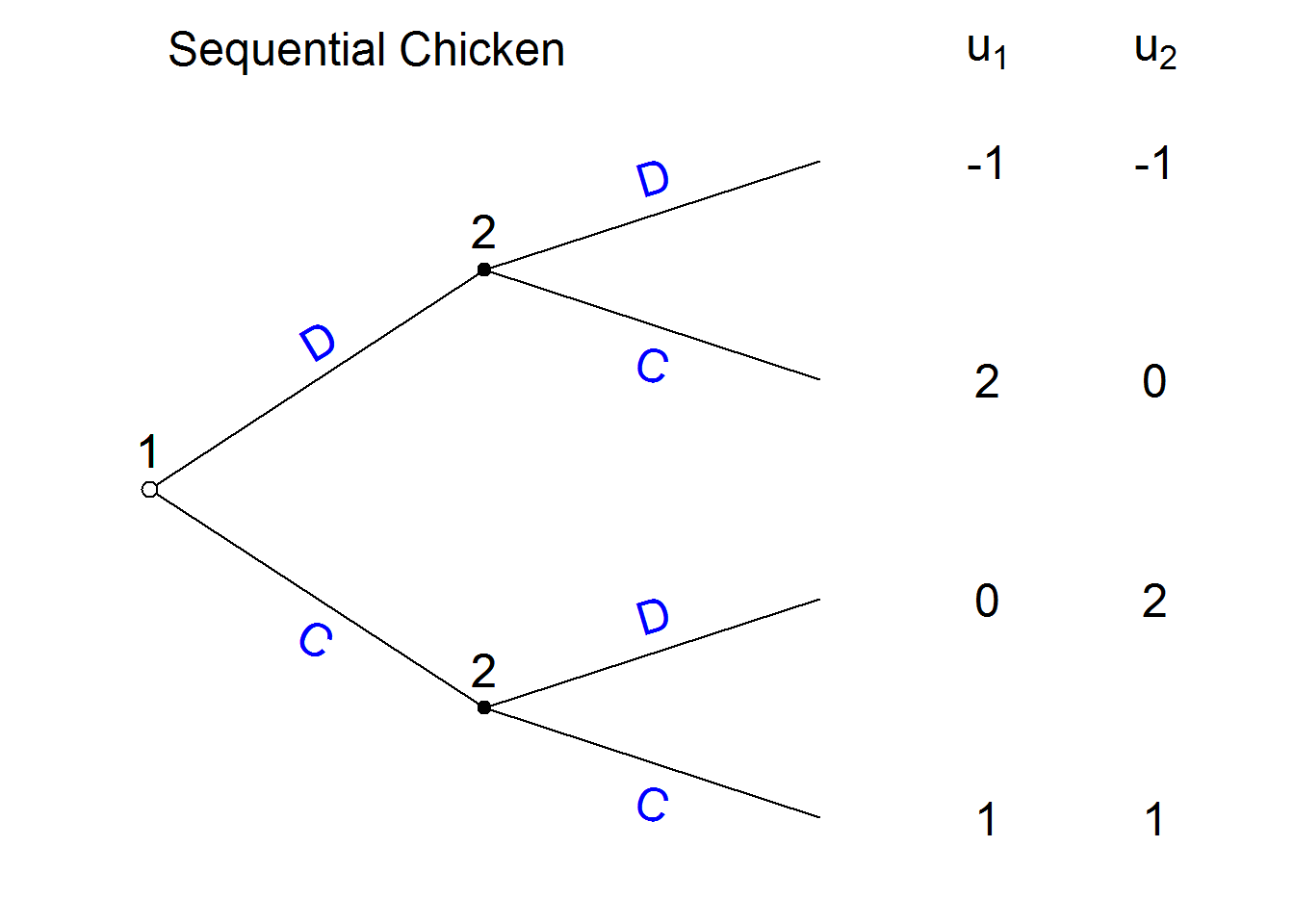The solution will however generally not appear for a non-generic game:

U[1,2] <- 0
gt_tree(H,U,P, title = "Non-generic", solution = TRUE)
##  "Game is not generic and solution not attempted."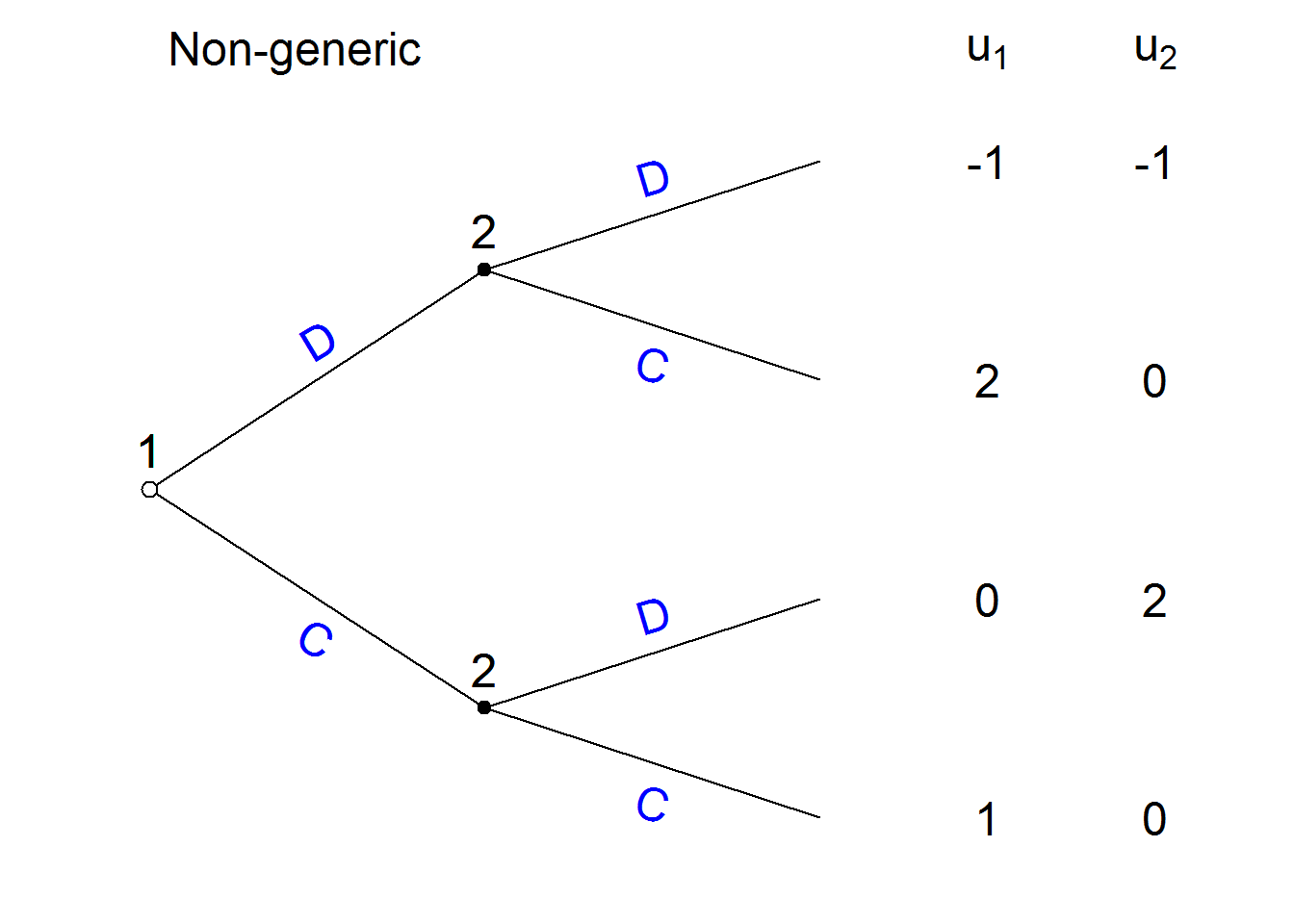Unless forced:

gt_tree(H,U,P, title = "Non-generic", solution = TRUE, force_solution = TRUE)
##  "Warning: Solution attempted even though game is not generic"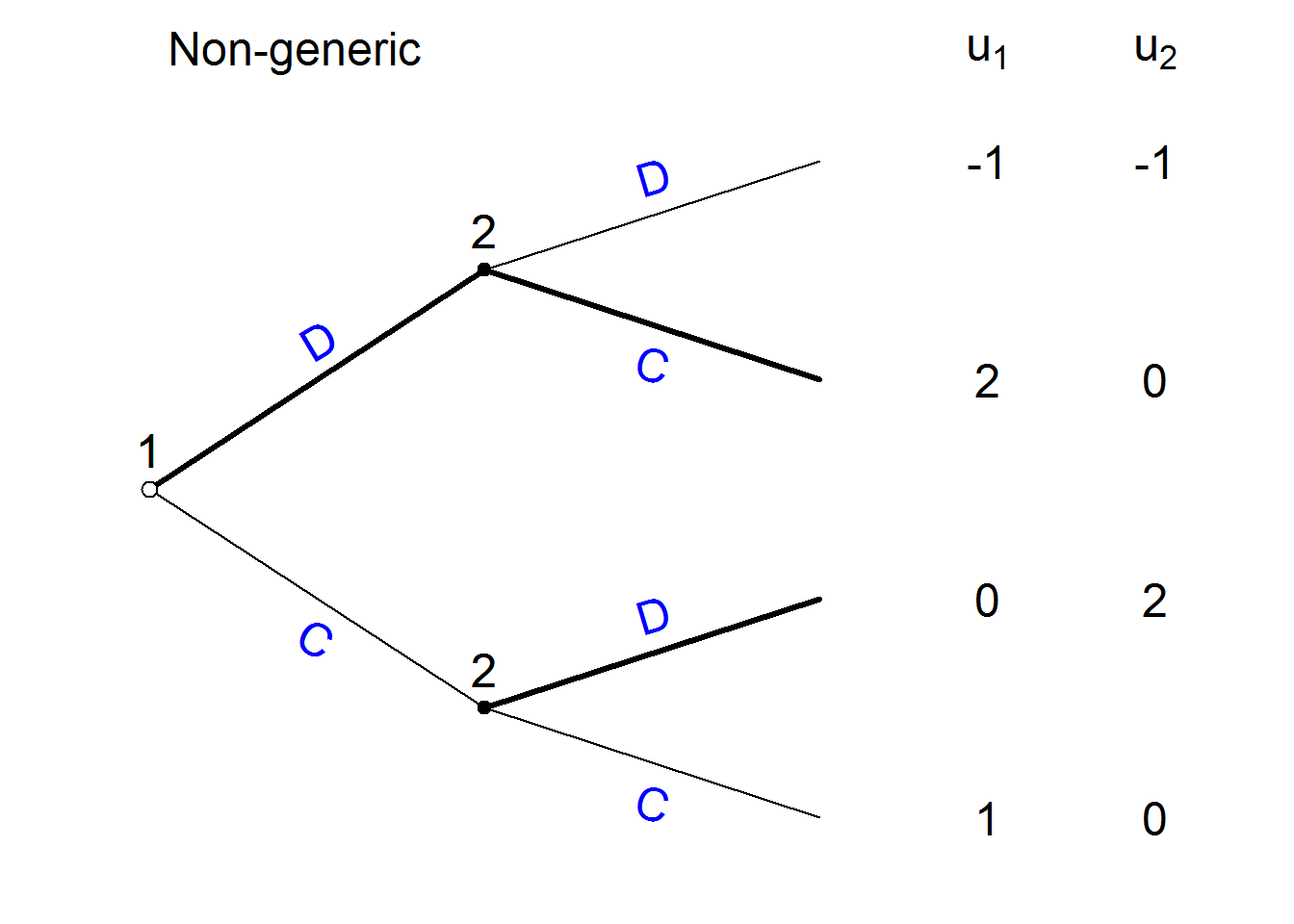This can however have undesireable consequences:

U[1,2] <- 2
gt_tree(H,U,P, title = "Non-generic", solution = TRUE, force_solution = TRUE)
##  "Warning: Solution attempted even though game is not generic"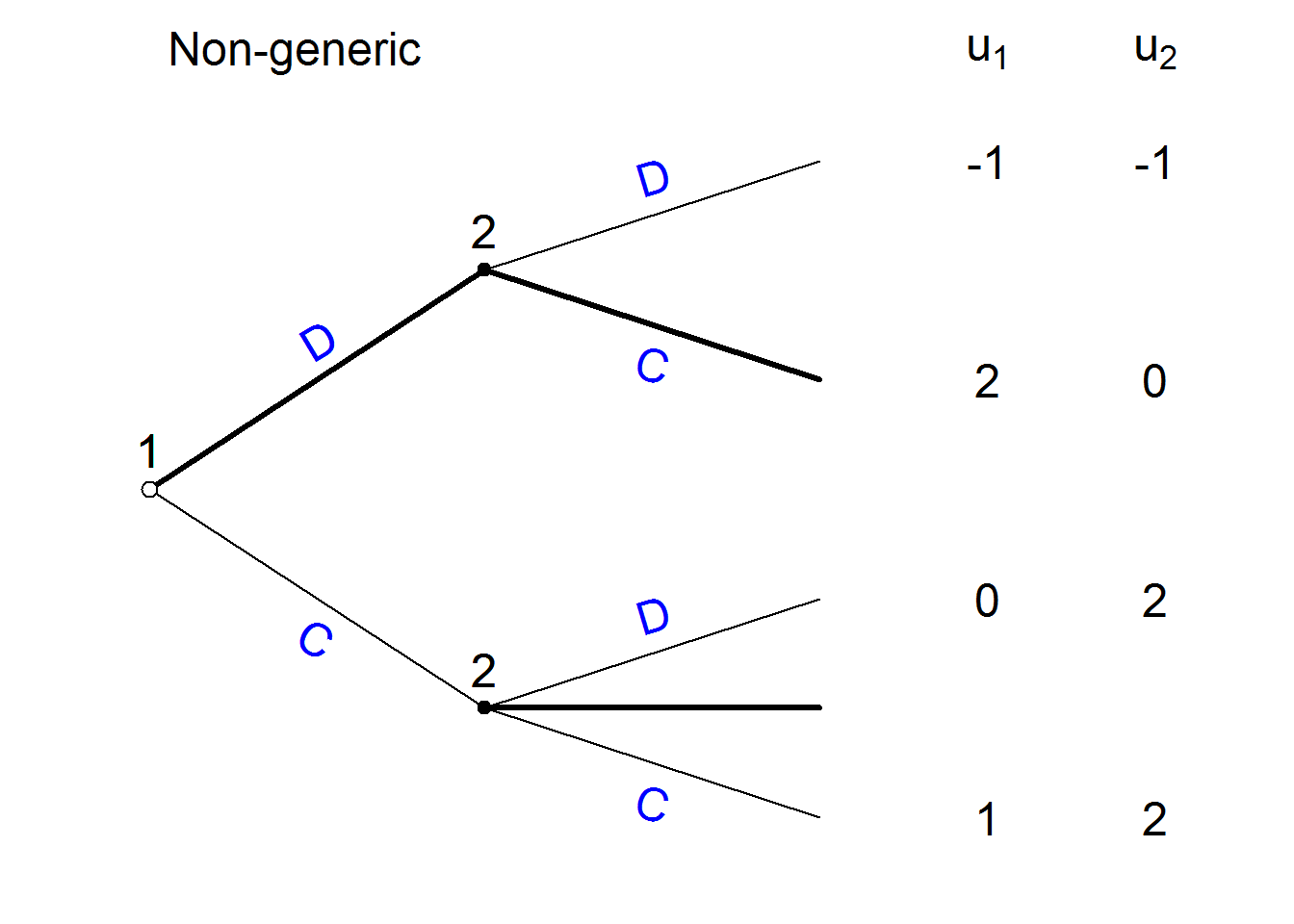# 5 Formatting

You can do a reasonable amount of formatting in terms of specifying sizes and colors. eg:

gt_tree(H,U,P,
title = "Sequential Chicken",
force_solution = TRUE,
player.names=c("Jack", "Jill"),
textsize  = 1,
titlesize = 2,
utextsize = 1.25,
btextsize = 1.5,
slopetext = FALSE,

branchcol = "orange",
actioncol = "black",
titlecol  = "grey",
playercol = "red",
solutioncol = "darkorange"
)
##  "Warning: Solution attempted even though game is not generic"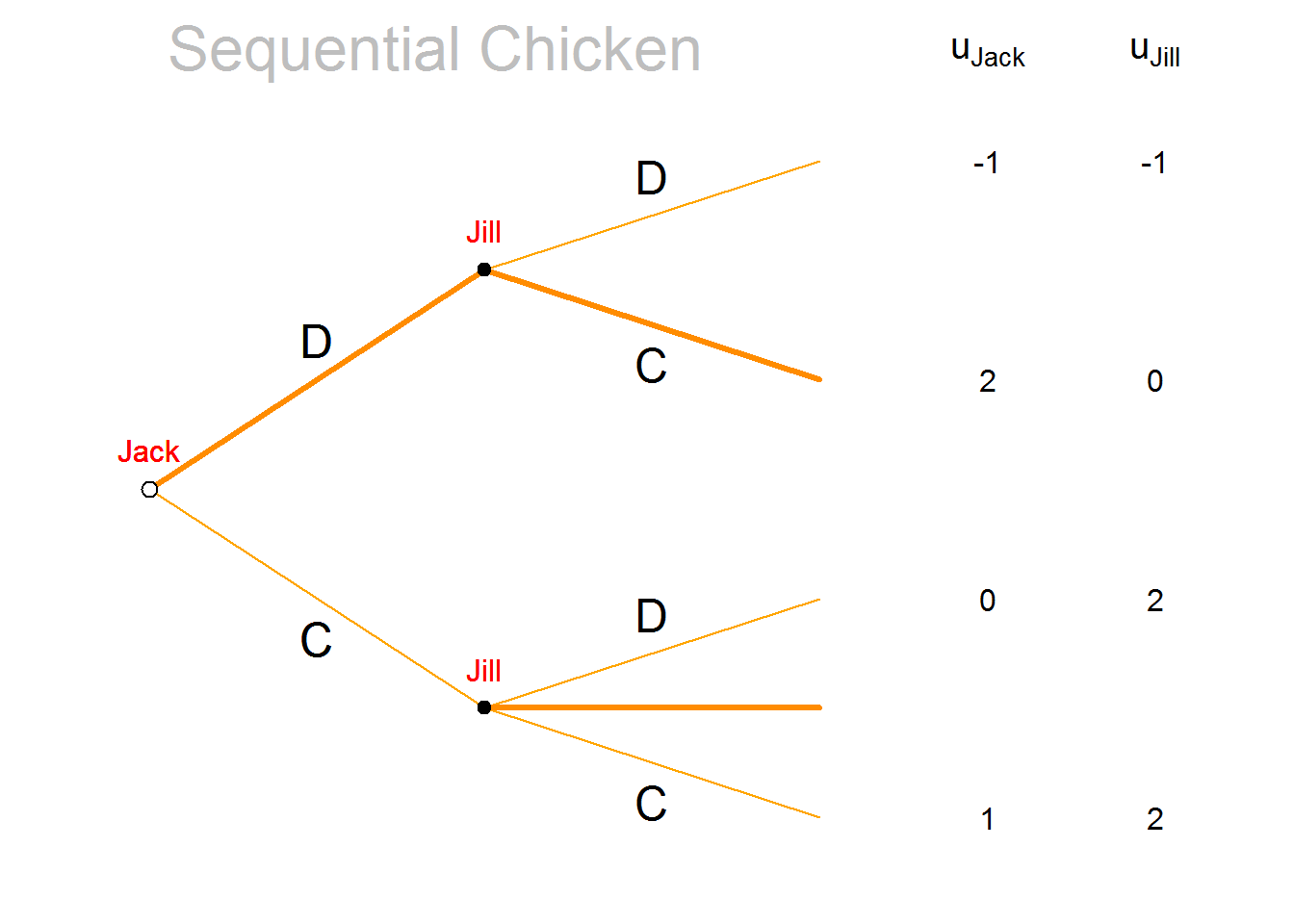# 6 Limitations

Besides being in alpha stage the main limitations of this function the fact that it cannot solve when there are multiple equilibria and payoff indifferences; and it cannot solve games of incomplete information. Even still it can be used to graph these and then then information sets and solutions can be superimposed.